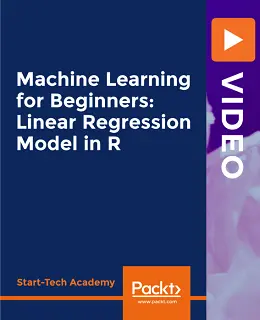# Machine Learning for Beginners: Linear Regression Model in R [Video]### Machine Learning for Beginners: Linear Regression Model in R [Video]

##### English | MP4 | AVC 1920×1080 | AAC 48KHz 2ch | 6h 13m | 1.91 GB eLearning | Skill level: All Levels

Machine Learning for Beginners: Linear Regression Model in R [Video]: Get up to speed with linear regression analysis for predictive machine learning and econometrics

Are you looking for a complete linear regression course that teaches you everything you need to create a linear regression model in R? This course covers the important aspects that you need to know to solve business problems through linear regression.

Although most courses only focus on teaching how to run the analysis, this course emphasizes what happens before and after analysis such as having the right data and performing preprocessing on it. You’ll also be able to judge how good your model is and interpret the results to help your business. As you progress, you will learn how to identify problems in your business and solve them using linear regression techniques. In addition to this, you’ll gain the knowledge you need to create a linear regression model in R and analyze its results.

• Identify the business problem and solve it using linear regression techniques
• Create a linear regression model in R and analyze its results
• Become well-versed with machine learning concepts
• Gain knowledge of data collection and data preprocessing for machine learning linear regression problems
• Explore advanced linear regression techniques using R’s glmnet package

By the end of this course, you will be equipped with the skills you need to effectively use linear regression for predictive machine learning and create robust models.